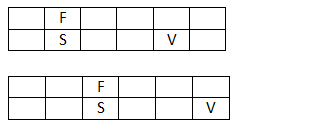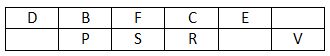### logical reasoning questions for cat: logical reasoning questions for cat that you should solve

Logical reasoning questions for cat exam cover a variety of topics. The most importantly tested topics are listed below:
• Games and Tournaments
• Input-Output or Sequential Output
• Logical Conditions (If this, then that)
• Logical Grouping (Questions based on Team Formation)
• Coding – Decoding
• Direction Sense
• Blood Relations and Family Tree
• Inequalities and Conclusions – Coded Inequalities
• Binary Logic – True / False statements based questions
• Linear and Circular Seating Arrangements
• Arrangement problems using Tables and Matrix
• Critical Path
• Cubes based problems
• Coin picking based problems
• Dice Related Problems
• Order and Ranking
• Quant Based LR Puzzles

Therefore, go through a variety of logical reasoning questions for cat to cover every single topic mentioned above and also cover a combination of two or more topics, for example Blood relations coupled with arrangement problems using tables and matrix.

Directions for the question set:
Study the following information to answer the given questions:
Twelve people are sitting in two parallel rows containing six people each, in such a way that there is an equal distance between adjacent persons. In row – 1 A, B, C, D, E and F are seated and all of them are facing south. In row – 2 P, Q, R, S, T and V are seeded and all of them are facing north. Therefore, in the given seating arrangement each member seated in a row faces another member of the other row.
V sits third to right of S. S faces F and F does not sit at any of the extreme ends of the line. D sits third to right of C. R faces C. The one facing E sits third to right of P. B and P do not sit at the extreme ends of the line. T is not an immediate neighbour of V and A is not an immediate neighbour of C.

Question 1: Who amongst the following faces D?
(a) T
(b) P
(c) Q
(d) R
(e) None of these

Question 2: Who amongst the following represent the people sitting at extreme ends of the rows?
(a) R, F
(b) T, A
(c) D, R
(d) C, Q
(e) S, A

Question 3: Four of the following five are alike in a certain way and thus form a group. Which is the one that does not belong to that group?
(a) B-T
(b) A-Q
(c) C-S
(d) F-P
(e) D-R

Question 4: Four of the following five are alike in a certain way and thus form a group. Which is the one that does not belong to that group?
(a) D
(b) S
(c) V
(d) T
(e) A

Question 5: How many individuals are seated between R and T?
(a) One
(b) Two
(c) Three
(d) Four
(e) None

Question 6: Who amongst the following faces Q?
(a) D
(b) B
(c) E
(d) A
(e) None of these

Question 7: Which of the following is true regarding B?
(a) B sits to the immediate left of C
(b) B faces Q
(c) B sits fourth from the extreme left end of the line
(d) D and F are immediate neighbours of B
(e) None is true

### Answers and Explanations: Click the down arrow to expand

Common Solution for the set: V sits third to right of S. S faces F and F does not sit at any of the extreme ends of the line. So two arrangements are possible.The one facing E sits third to right of P. B and P do not sit at the extreme ends of the line. This removes one arrangement so we left with 2nd one.

Also, D sits third to right of C. R faces C. b will also do not sit at any end so B will sit between D & F.T is not an immediate neighbour of V so, T will sit near P and A is not an immediate neighbour of C. So, A will sit near E.Answer 1: (a) T faces D Correct option is (a)

Answer 2: (b) The correct option is (b)

Answer 3: (e) D-R is different. In the rest the first person to the right of the person who is opposite to the second person. Correct option is (e).

Answer 4: (b) In this case, S is the odd one out; all the others are seated in the extreme ends. SO option (b) is correct.

Answer 5: (b) There are two people between R and T. Correct option is (b)

Answer 6: (c) E faces Q. Correct option is (c).

Answer 7: (d) Correct option is (d).

Extra tips for logical reasoning questions for cat:
• Solve as many as logical reasoning questions for cat exam as you can to cover all the topics.
• Ensure that you stick to time limits right through your practice. This will improve your speed.
• logical reasoning questions for cat exam from various topics will help you sharpen your analytical abilities therefore solve all levels of questions.